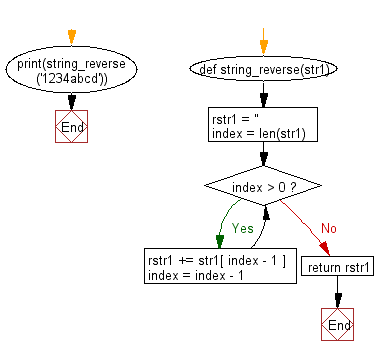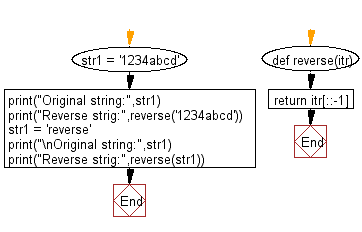﻿ Python Exercise: Reverse a string - w3resource# Python Exercise: Reverse a string

## Python Functions: Exercise-4 with Solution

Write a Python program to reverse a string.

Sample String: "1234abcd"
Expected Output: "dcba4321"

Sample Solution-1:

Python Code:

``````def string_reverse(str1):

rstr1 = ''
index = len(str1)
while index > 0:
rstr1 += str1[ index - 1 ]
index = index - 1
return rstr1
print(string_reverse('1234abcd'))
```
```

Sample Output:

```dcba4321
```

Pictorial presentation:Flowchart:## Visualize Python code execution:

The following tool visualize what the computer is doing step-by-step as it executes the said program:

Sample Solution-2:

Python Code:

``````def reverse(itr):
return itr[::-1]

str1 = '1234abcd'
print("Original string:",str1)
print("Reverse strig:",reverse('1234abcd'))
str1 = 'reverse'
print("\nOriginal string:",str1)
print("Reverse strig:",reverse(str1))
```
```

Sample Output:

```Original string: 1234abcd
Reverse strig: dcba4321

Original string: reverse
Reverse strig: esrever
```

Flowchart:## Visualize Python code execution:

The following tool visualize what the computer is doing step-by-step as it executes the said program:

Python Code Editor:

Have another way to solve this solution? Contribute your code (and comments) through Disqus.

What is the difficulty level of this exercise?

Test your Programming skills with w3resource's quiz.

﻿

## Python: Tips of the Day

Decapitalizes the first letter of a string:

Example:

```def tips_decapitalize(s, upper_rest=False):
return s[:1].lower() + (s[1:].upper() if upper_rest else s[1:])
print(tips_decapitalize('PythonTips'))
print(tips_decapitalize('PythonTips', True))
```

Output:

```pythonTips
pYTHONTIPS
```